# Forecasting by Regression¶

Sept. 14, 2018

Machine learning and time series analysis are separate disciplines. One might wonder whether machine learning techniques can be applied to time series analysis. The answer is definitely yes. In this post I will explore how simple machine learning techniques such as linear regression and tree based methods can be used to make time series forecast. The more experienced a model builder is, the more she will realize that there is no "free lunch" type of model. The models we use are simply tools--It is up to the us to decide how to utilize them. Sometimes, a simple model does better than fancy ones.

The time series dataset we will be using for this analysis is the weekly flu data freely available on CDC's website. To download it, click on Download Data, and check the box Select All for season selection. Basically, we are going to download weekly flu data from 1997 to 2018. The data file is named ILInet.csv. The acronym "ILI" is short for "Influenza-Like Illness". Next, let's import some Python libraries.

In :
import numpy as np
import matplotlib.pyplot as plt
import pandas as pd
import seaborn as sns; sns.set()
import copy
import itertools
from xgboost import XGBRegressor
from sklearn.linear_model import LinearRegression
%matplotlib inline


We are going to read the file ILInet.csv, and extract the columns ILITOTAL and WEEK. The ILITOTAL column contains the total count of ILI cases, and WEEK is the week number. For the ease of calculation, we are going to drop all the 53rd week. (So we assume that every year consistantly contains 52 weeks.)

In :
# Read the data

# Drop all the 53rd week values and reset the index
flu = flu[flu['WEEK'] != 53].reset_index()[['ILITOTAL', 'WEEK']]

# Check the first 5 rows of the data

Out:
ILITOTAL WEEK
0 570 40
1 615 41
2 681 42
3 653 43
4 700 44

To get a feel of the time series, let's plot it! Plotting is always the first step before any data analysis.

In :
plt.rcParams['figure.figsize'] = [10, 5]
flu['ILITOTAL'].plot()

Out:
<matplotlib.axes._subplots.AxesSubplot at 0x103915860>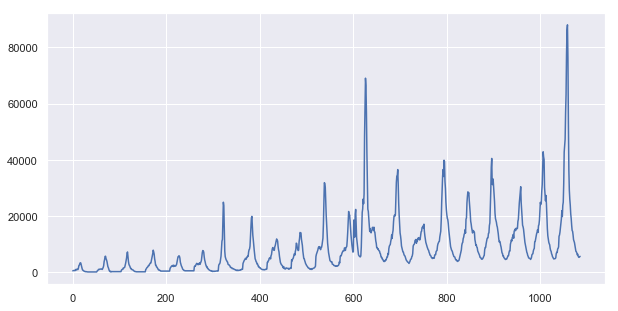Just by looking at this time series, we see a strong seasonal patter and a strong positive trend. So immediately we know that the time series is highly correlated with the season values (in our case, the week values), as well as the time values (the index). So our machine learning model should include both as the predictors!

Furthermore, we can also use the past values as the predictor. To accomplish that, all we need to do is to shift our time series several steps back, and combine all shifted series into a single dataframe. Finally we remove the initial rows that contains the NaN values. This is illustrated by the following figure.In addition to the shifted value, we will also add the week number as well as the index as two additional predictors. So our final dataframe looks like the following.Notice that once we hit week 52, we will restart at 1. This can be accomplished using the transform function below.

In :
# define a function that transforms time series into training data

def transform(data, shift_range=1):

"""
data is a data frame with two columns: ILITOTAL and WEEK
original dimension: (m, 2)
returns a dataframe with shifted variables and index as features
new dimension: (m, 2+shift_range)
"""

ilitotal = data[['ILITOTAL']]

week = data[['WEEK']]

# Form a list of columns of the final dataframe
data = [ilitotal, ]

for i in range(shift_range):

# Add "NaN" values to the shifted ilitotal
shift = pd.DataFrame(np.full([i+1, 1], np.nan), columns=['ILITOTAL'])

ilitotal_shifted = shift.append(ilitotal, ignore_index=True, sort=False)

data.append(ilitotal_shifted)

# Extending the week values ahead (mod 52)
week_extended = pd.DataFrame({'WEEK':[(week.iloc[-1]+i)%52+1 for i in range(shift_range)]})

week = week.append(week_extended, ignore_index=True, sort=False)

data.append(week)

# Forming the new data frame
data = pd.concat(data, axis=1)

data.columns = ['count'] + ['shift'+str(i) for i in range(shift_range)] + ['week']

# Adding the index values as a variable to account for the trend
data['index']=data.index

# Crop missing values in beginning rows due to the shift
data= data.iloc[shift_range:,:]

return data


Let's test this function.

In :
# Define a simple time series of 1-5
df = pd.DataFrame({'ILITOTAL': [1, 2, 3, 4, 5], 'WEEK': [47, 48, 49, 50, 51]})
df

Out:
ILITOTAL WEEK
0 1 47
1 2 48
2 3 49
3 4 50
4 5 51
In :
df_shift = transform(df, shift_range=3)
df_shift

Out:
count shift0 shift1 shift2 week index
3 4.0 3.0 2.0 1.0 50 3
4 5.0 4.0 3.0 2.0 51 4
5 NaN 5.0 4.0 3.0 52 5
6 NaN NaN 5.0 4.0 1 6
7 NaN NaN NaN 5.0 2 7

Using simple case above as an example, the next step is to feed the first two rows of the data frame (without NaN values) into a machine learning algorithm and then use model to sequentially fill out the rest of the table. The process is illustrated in the figure below.For instance, we first use the predictors in the third row $[5, 4, 3, 52, 5]$ to predict the value of pred 1. Once we have pred 1, we can use the fourth row $[\text{pred 1}, 5, 4, 1, 6]$ as inputs to predict pred 2. This way, we can sequentially fill out the missing triangle of NaN values.

What if we want to make predictions farther down into the future? This can be easily achieved by first appending the dataframe with NaN values and then repeat the same process. Suppose we want to predict three additional weeks into the future. The process is illustrated in the figure below. The highlighted cells represent the additional future values.To accomplish that, we first define a function transform_forecast that further augments the dataframe to accomodate higher prediction_range.

In :
def transform_forecast(data, prediction_range, shift_range):

# Defining the difference between prediction range and shift range
diff = prediction_range - shift_range

# If the prediction range is less than the shifted value, crop the data frame
if diff < 0:

data = data.iloc[:diff]

# If we want to predict farther into the future, we append more rows to the dataframe.
elif diff > 0:

# Extending the first (shift_range+1) columns with an array of "NaN" values
shift_diff = np.full((diff, shift_range+1), np.nan)

# Extending the week values (mod 52)
week_diff = np.array([[(data['week'].iloc[-1]+i)%52+1 for i in range(diff)]])

# Extending the index values
index_diff = np.array([[data['index'].iloc[-1]+i+1 for i in range(diff)]])

# Combine all extended arrays
data_diff = pd.DataFrame(np.hstack((shift_diff,
week_diff.reshape(week_diff.shape,-1),
index_diff.reshape(index_diff.shape, -1))))

data_diff.columns = ['count'] + ['shift'+str(i) for i in range(shift_range)] + ['week', 'index']

data = data.append(data_diff, ignore_index=True, sort=False)

return data

In [ ]:
df_forecast = transform_forecast(df_shift, prediction_range=6, shift_range=3)
df_forecast


Now we are going to fit a linear regression to our model. To illustrate using the simple example above, we are going to use the 0th row as the training set, and first row as the test set. To do so we need to define a fit function.

In :
def fit_data(data, model="linear_regression", shift_range=3, test_size = 1, alpha=1):

# Using past values, week, and index as predictors
data = transform(data, shift_range)

# Selecting the machine learning model
if model == "xgboost":

model = XGBRegressor(min_child_weight=alpha)

elif model == "linear_regression":

model = LinearRegression(normalize=True)

# Selecting the input variable in the training set

X = data[:-shift_range-test_size][['shift'+str(i) for i in range(shift_range)] +
['week', 'index']]

# Selecting the output variable in the training set
y = data[:-shift_range-test_size]['count']

# Fitting the model
model.fit(np.array(X), y)

return model, data

In :
mymodel, _ = fit_data(df)
mymodel

Out:
LinearRegression(copy_X=True, fit_intercept=True, n_jobs=1, normalize=True)

Having trained our model, we can now fill out the rest of the NaN values.

In :
def forecast_data(data, model, prediction_range=6, shift_range=3, test_size=1, print_process = False):

data = transform_forecast(data, prediction_range, shift_range)

# Converting to numpy array for faster computation
data = np.array(data)

m, n = data.shape

# Obtain feature variables
X = data[:,range(1, n)]

# Response variable
y = data[:, 0].reshape(m, 1)

# Making a deep copy of X and y
y_orig = copy.deepcopy(y)

if print_process == True:

print("------------")
print("Initial data")
print("------------")
print(X)

# Making predictions to the test set by sequentially filling the matrix
for i in range(prediction_range+test_size):

# Note that the prediction starts at the first value of the test set.
prediction = model.predict([X[-prediction_range-test_size+i, :]])

y[-prediction_range-test_size+i, 0] = prediction

# Diagonally fill in the predicted value
for j in range(shift_range):

try:

# Prevent negative index to affect start values
if -prediction_range-test_size+i+j+1 < 0:

X[-prediction_range-test_size+i+j+1, j] = prediction

else:

break

except IndexError:

break

if print_process == True:

print("------------")
print("Iteration {}".format(i+1))
print("------------")
print(X)

# Making predictions to the rest of the training set (for a visual check of the model's bias)
for i in range(m-prediction_range-test_size):

prediction = model.predict([X[i, :]])

y[i, 0] = prediction

return X, y, y_orig, m


The process of the algorithm is illustrated below. Note that since we only have one data point, the regression will only predict 4 as the output.

In :
X, y, y_orig, m = forecast_data(df_shift, mymodel, print_process=True)

------------
Initial data
------------
[[ 3.  2.  1. 50.  3.]
[ 4.  3.  2. 51.  4.]
[ 5.  4.  3. 52.  5.]
[nan  5.  4.  1.  6.]
[nan nan  5.  2.  7.]
[nan nan nan  3.  8.]
[nan nan nan  4.  9.]
[nan nan nan  5. 10.]]
------------
Iteration 1
------------
[[ 3.  2.  1. 50.  3.]
[ 4.  3.  2. 51.  4.]
[ 4.  4.  3. 52.  5.]
[nan  4.  4.  1.  6.]
[nan nan  4.  2.  7.]
[nan nan nan  3.  8.]
[nan nan nan  4.  9.]
[nan nan nan  5. 10.]]
------------
Iteration 2
------------
[[ 3.  2.  1. 50.  3.]
[ 4.  3.  2. 51.  4.]
[ 4.  4.  3. 52.  5.]
[ 4.  4.  4.  1.  6.]
[nan  4.  4.  2.  7.]
[nan nan  4.  3.  8.]
[nan nan nan  4.  9.]
[nan nan nan  5. 10.]]
------------
Iteration 3
------------
[[ 3.  2.  1. 50.  3.]
[ 4.  3.  2. 51.  4.]
[ 4.  4.  3. 52.  5.]
[ 4.  4.  4.  1.  6.]
[ 4.  4.  4.  2.  7.]
[nan  4.  4.  3.  8.]
[nan nan  4.  4.  9.]
[nan nan nan  5. 10.]]
------------
Iteration 4
------------
[[ 3.  2.  1. 50.  3.]
[ 4.  3.  2. 51.  4.]
[ 4.  4.  3. 52.  5.]
[ 4.  4.  4.  1.  6.]
[ 4.  4.  4.  2.  7.]
[ 4.  4.  4.  3.  8.]
[nan  4.  4.  4.  9.]
[nan nan  4.  5. 10.]]
------------
Iteration 5
------------
[[ 3.  2.  1. 50.  3.]
[ 4.  3.  2. 51.  4.]
[ 4.  4.  3. 52.  5.]
[ 4.  4.  4.  1.  6.]
[ 4.  4.  4.  2.  7.]
[ 4.  4.  4.  3.  8.]
[ 4.  4.  4.  4.  9.]
[nan  4.  4.  5. 10.]]
------------
Iteration 6
------------
[[ 3.  2.  1. 50.  3.]
[ 4.  3.  2. 51.  4.]
[ 4.  4.  3. 52.  5.]
[ 4.  4.  4.  1.  6.]
[ 4.  4.  4.  2.  7.]
[ 4.  4.  4.  3.  8.]
[ 4.  4.  4.  4.  9.]
[ 4.  4.  4.  5. 10.]]
------------
Iteration 7
------------
[[ 3.  2.  1. 50.  3.]
[ 4.  3.  2. 51.  4.]
[ 4.  4.  3. 52.  5.]
[ 4.  4.  4.  1.  6.]
[ 4.  4.  4.  2.  7.]
[ 4.  4.  4.  3.  8.]
[ 4.  4.  4.  4.  9.]
[ 4.  4.  4.  5. 10.]]


Finally, we need a measure of how well the model is doing. To do so, we can use final portions of the time series as the test set, and the rest as the training set. An obvious accuracy measure is the Root Mean Squared Error, defined by

$$\text{RMSE} = \sqrt{\frac{1}{n}\sum_{t=1}^n (y_t - \widehat{y}_{t})^2} \tag{1}$$

where $y_1,y_2,..., y_n$ are the true values of the time series in the test set, and $\widehat{y}_t$ are the predicted values. Let's put everything into a model!

In :
class tslearn:

def __init__(self, shift_range = 6):

self.shift_range = shift_range

self.data = None

self.model = None

self.test_size = None

self.score = None

# Fitting the model with data
def fit(self, data=flu, model="linear_regression", test_size = 1, alpha=1):

self.test_size = test_size

self.model, self.data = fit_data(data, model, self.shift_range, test_size, alpha)

return self

# Making forecasts
def forecast(self, prediction_range=1, plot=True):

X, y, y_orig, m = forecast_data(self.data, self.model, prediction_range, self.shift_range, self.test_size)

# The original time series
y_orig = pd.DataFrame(y_orig, columns=['count'])

# The original test set
y_orig_test = y_orig['count'].iloc[-prediction_range-self.test_size:-prediction_range]

# The fitted values of the entire time series
y = pd.DataFrame(y, columns=['count'])

# The fitted values to the test set
y_test = y['count'].iloc[-prediction_range-self.test_size:-prediction_range]

if plot == True:

# The fitted values to the training set
y_train = y['count'].iloc[:-prediction_range-self.test_size+1]

# The forecasting values
y_forecast = y['count'].iloc[-prediction_range-1:]

# Plotting predictions
y_train.plot(color='orange', lw=3)

y_test.plot(color='orange', lw=5)

y_forecast.plot(color='red', lw=5)

# Plotting original time series
y_orig['count'].plot(color='b')

# Drawing a vertical line to denote the start of the test set
plt.axvline(x=m-prediction_range-self.test_size, color='r', ls=':')

# Calculate the Root Mean Squared Error of the model
self.score = np.sqrt(np.mean((y_orig_test - y_test)**2))

# For illustration, returning score is sufficient
return self.score


We are now ready to forecast! Let's start with a simple linear regression using the past week's data as predictor. We are predicting three years ahead into the future.

In :
# Define a function that plots the forecasts
def model_plot(data, model="linear_regression", shift_range=1, test_size=250, prediction_range=52*3, alpha=1):

tsmodel = tslearn(shift_range = shift_range)

tsmodel.fit(data, model, test_size, alpha=alpha)

score = tsmodel.forecast(prediction_range, plot=True)

plt.title("model = {}, shift_range = {}, score = {}".format(model, shift_range, score))

In :
model_plot(flu)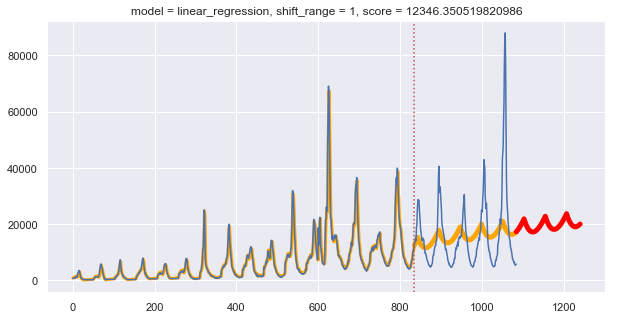By looking at the plot, we know that our model has a low bias and a high variance. There is no problem for the model to fit the training set, but the test set performance is poor. We can increase the parameter shift_range.

In :
model_plot(flu, shift_range=100)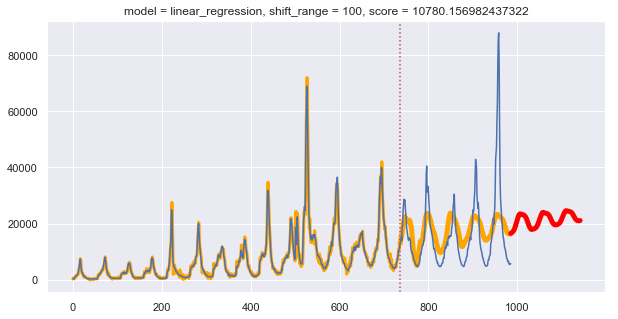Next we look at the predictions from the XGBoost. It is one of the "out-of-the-box" machine learning algorithms that works with very large data sets.

In :
model_plot(flu, model="xgboost", shift_range=90, alpha=2)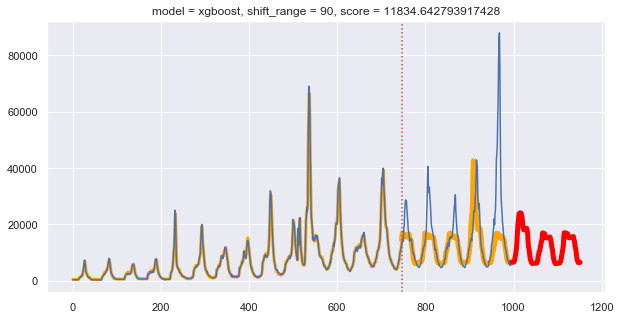It looks like that the boosting algorithm is able to predict certain high peak but failed to predict the last high peak. Compared to linear regression, the boosting model produces results that are less "naive". It will be a powerful method to try when we build more sophisticated models.

The catch is, there are a lot of parameters one can tune. So we can use the following GridSearch function to determine the optimal parameters.

In :
def GridSearch(model, parameters, plot=True):

"""
parameters: must be a dictionary of the form
{"model: [], "shift": []}
"""
scores, models, shifts = [], [], []

for param in itertools.product(*parameters.values()):

tsmodel = model(shift_range=param)

tsmodel.fit(flu, model=param, test_size=250)

score = tsmodel.forecast(prediction_range=53*3, plot=False)

scores.append(score)

models.append(param)

shifts.append(param)

df = pd.DataFrame({'model': models, 'shift': shifts, 'score': scores})

if plot == True:

sns.lineplot(data=df, x='shift', y='score', hue='model')

min_index = scores.index(min(scores))

return models[min_index], shifts[min_index], scores[min_index]


We are ready to tune the parameters for the linear regression model.

In :
parameters = {"model": ["linear_regression"],
"shift": [5*i for i in range(1, 45)]}

In :
GridSearch(tslearn, parameters, plot=True)

Out:
('linear_regression', 195, 9288.682706396216)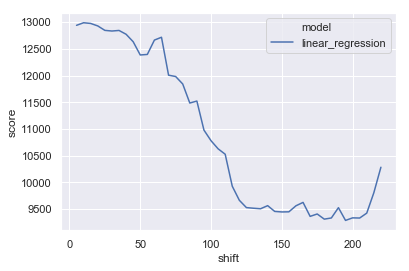So we see that the model seems to improve dramatically past the point when shift_size exceeds 125. We check the optimual value of 195.

In :
model_plot(flu, shift_range=195)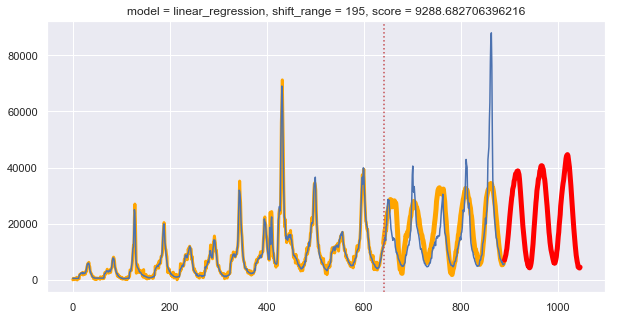The model we built is clearly far from perfect. The linear regression model is best in capturing seasonality and trend. As we include more meaningful predictors, we can try more flexible models.